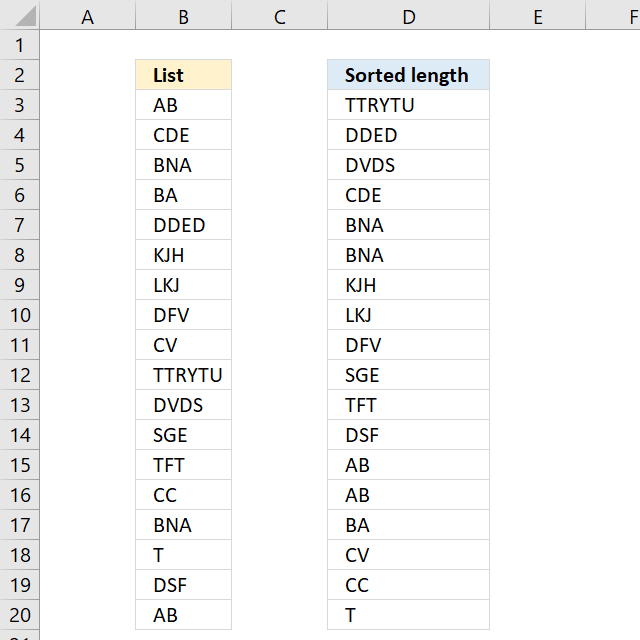Author: Oscar Cronquist Article last updated on December 05, 2018Array formula in B2:

=INDEX(\$B\$3:\$B\$20, MATCH(LARGE(LEN(\$B\$3:\$B\$20), ROWS(\$A\$1:A1)), LEN(\$B\$3:\$B\$20)*(COUNTIF(\$F\$2:F2, \$B\$3:\$B\$20)<COUNTIF(\$B\$3:\$B\$20, \$B\$3:\$B\$20)), 0))

copied down as far as needed.

To enter an array formula, type the formula in a cell then press and hold CTRL + SHIFT simultaneously, now press Enter once. Release all keys.

The formula bar now shows the formula with a beginning and ending curly bracket telling you that you entered the formula successfully. Don't enter the curly brackets yourself.

### Explaining formula in cell B2

#### Step 1 - Calculate the k-th largest string length

In order to return a new value in each cell, the formula uses an expanding cell reference to return the next largest value. The LEN function returns the character length of a value, the ROWS function returns the number of rows in a cell reference. The LARGE function returns the k-th largest value. LARGE( array, k)

LARGE(LEN(\$B\$3:\$B\$20), ROWS(\$A\$1:A1))

becomes

LARGE(LEN(\$B\$3:\$B\$20), 1)

becomes

LARGE({2; 3; 3; 2; 4; 3; 3; 3; 2; 6; 4; 3; 3; 2; 3; 1; 3; 2}, 1)

and returns 6.

#### Step 2 - Check previously displayed values in cells above

The COUNTIF function counts cells in cell range based on a condition or criteria. The first argument contains an expanding cell reference that lets the formula keep track of already shown values.

COUNTIF(\$F\$2:F2, \$B\$3:\$B\$20)

becomes

COUNTIF("Sorted length",{"AB"; "CDE"; "BNA"; "BA"; "DDED"; "KJH"; "LKJ"; "DFV"; "CV"; "TTRYTU"; "DVDS"; "SGE"; "TFT"; "CC"; "BNA"; "T"; "DSF"; "AB"})

and returns

{0;0;0;0;0;0;0;0;0;0;0;0;0;0;0;0;0;0}

#### Step 3 - Check if the count of prior values are less than the total count of each value

(COUNTIF(\$D\$2:D2,\$B\$3:\$B\$20)<COUNTIF(\$B\$3:\$B\$20,\$B\$3:\$B\$20)

becomes

{0; 0; 0; 0; 0; 0; 0; 0; 0; 0; 0; 0; 0; 0; 0; 0; 0; 0}<{2; 1; 2; 1; 1; 1; 1; 1; 1; 1; 1; 1; 1; 1; 2; 1; 1; 2}

and returns

{TRUE; TRUE; TRUE; TRUE; TRUE; TRUE; TRUE; TRUE; TRUE; TRUE; TRUE; TRUE; TRUE; TRUE; TRUE; TRUE; TRUE; TRUE}.

#### Step 4 - Match length to array

The MATCH function returns the relative position in a cell range or array of a given value.

MATCH(LARGE(LEN(\$B\$3:\$B\$20), ROWS(\$A\$1:A1)), LEN(\$B\$3:\$B\$20)*(COUNTIF(\$F\$2:F2, \$B\$3:\$B\$20)<COUNTIF(\$B\$3:\$B\$20, \$B\$3:\$B\$20)), 0)

becomes

MATCH(6, LEN(\$B\$3:\$B\$20)*(COUNTIF(\$F\$2:F2, \$B\$3:\$B\$20)<COUNTIF(\$B\$3:\$B\$20, \$B\$3:\$B\$20)), 0)

becomes

MATCH(6, LEN(\$B\$3:\$B\$20)*{TRUE; TRUE; TRUE; TRUE; TRUE; TRUE; TRUE; TRUE; TRUE; TRUE; TRUE; TRUE; TRUE; TRUE; TRUE; TRUE; TRUE; TRUE}, 0)

becomes

MATCH(6, {2; 3; 3; 2; 4; 3; 3; 3; 2; 6; 4; 3; 3; 2; 3; 1; 3; 2}*{TRUE; TRUE; TRUE; TRUE; TRUE; TRUE; TRUE; TRUE; TRUE; TRUE; TRUE; TRUE; TRUE; TRUE; TRUE; TRUE; TRUE; TRUE}, 0)

becomes

MATCH(6, {2; 3; 3; 2; 4; 3; 3; 3; 2; 6; 4; 3; 3; 2; 3; 1; 3; 2}, 0)

and returns 10.

#### Step 5 - Return value

The INDEX function returns a value based on row number (and column number if needed)

INDEX(\$B\$3:\$B\$20, MATCH(LARGE(LEN(\$B\$3:\$B\$20), ROWS(\$A\$1:A1)), LEN(\$B\$3:\$B\$20)*(COUNTIF(\$F\$2:F2, \$B\$3:\$B\$20)<COUNTIF(\$B\$3:\$B\$20, \$B\$3:\$B\$20)), 0))

becomes

INDEX(\$B\$3:\$B\$20, 10)

amd returns TTRYTU in cell D3.

#### Download Excel *.xlsx file

Sort text based on length.xlsx